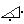# Number XY Angle node

Left Toolbar >Math Nodes >Number XY Angle

The Number XY Angle node allows you to calculate the angle of the vector defined by point xy with the x axis.

## Procedure

### To calculate a Number XY angle in the node graph:

1. On the left toolbar choose Math Nodes> Number XY Angle2. Drag the Number XY Angle icon into the Node Graph.3. Connect the Output: Result.

## Inputs

X

X position. A NUMBER type of value.

Y

Y position. A NUMBER type of value.

## Outputs

Result

The angle of point xy in degrees.

## Attributes PanelX

X value. This attribute is exposed as input by default. It can be defined in the attributes panel as well.

Y

Y Value. This attribute is exposed as input by default. It can be defined in the attributes panel as well.

Math Nodes

Main Page